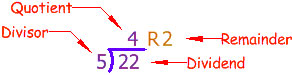Personal tools

You are here: Home Basic Math Definitions

# Basic Math Definitions

### The Basic Operations

In math problems there are many ways of saying the same thing. We have collected some of them here

 Symbol Words Used + Add, Addition, Sum, Plus, Increase, Total - Subtract, Subtraction, Minus, Difference, Decrease, Take Away, Deduct x Multiply, Multiplication, Product, By, Times, Lots Of ÷ Divide, Division, Quotient, Goes Into, How Many Times

### Division

Division has its own special words to remember.

Let's take the simple problem of dividing 22 by 5. The answer is 4, with 2 left over. Here we illustrate the important words:Which is the same as:Document Actions